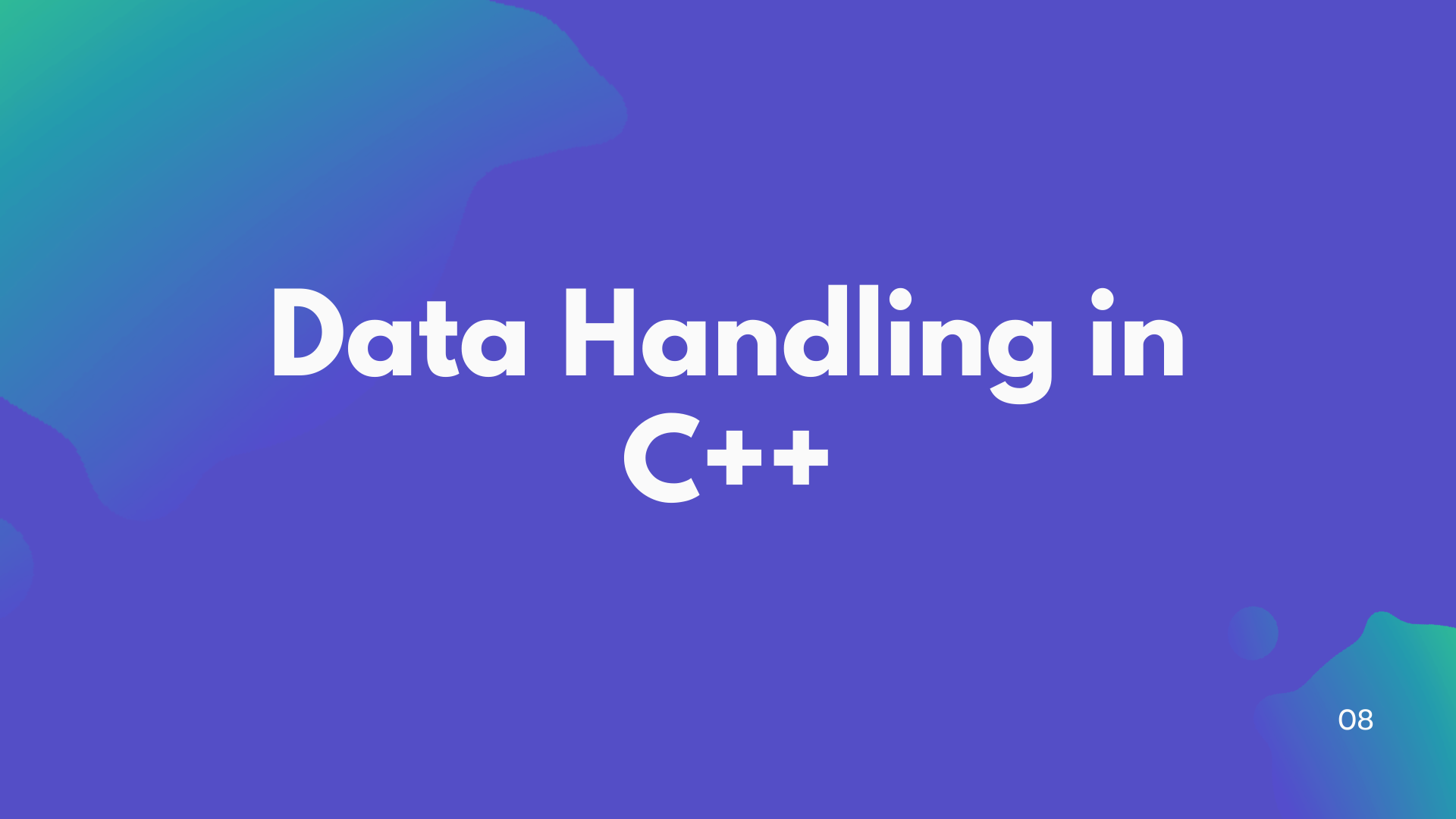# Data Handling in C++ (Part 1)

## In all programming languages, there are predefined sets of data types for handling the data. In this blog, we are going to learn about data handling in C++.## Introduction

In every programming language, there are some fundamentals we need to know even before writing the simplest programs. Now onwards in this series, of course, we are going to learn about two such fundamentals data types and variables in C++.

C++ comes up with a predefined set of data types for handling data in the program. The data types can be of two types fundamental and derived. The derived data types in C++ come in two groups that are built-in types and user-defined types. Data can be stored using any of these data types.

## Concepts of Data Types

In computers, data can be of many types e.g., character, integer, real, string, etc. Anything enclosed in the single quotes is the type of character data in C++. Numbers without fractional value are integer data. Number with fraction represent real data and anything enclosed in the double quote is a string. Since data is many types so all programming languages provide ways to handle such data.

In C++ data types can be divide into two parts :

1.  Fundamental Types
2. Derived Types

There are five fundamental data types in C++: char, int, float, double, and void that represent the character, integer, floating-point, double floating-point, and null value data respectively. These are the data types as per the original first edition of C++. The C++ specifications are per the latest standard will be covered in future blogs.

Derived types constructed from the fundamental types are : array, functions, pointer, references, constants, classes, structures, unions, and enumerations.

As we know now that there are five fundamental data types in C++. All other data types in C++ are based upon one of these fundamental data types which are known as derived data types. The size and range of these data types vary with each processor type and with the implementation of the C++ compiler. A character is generally 1 byte and an integer is 2 byte, but we can not make this assumption if you want your programs to be portable to the widest range of computers. We will discuss each type of data types in detail in the next blog.

Now, We will stop here in this tutorial and will study types of data types in detail in the next tutorial. If you have any query drop your comment in the comment section.0000000